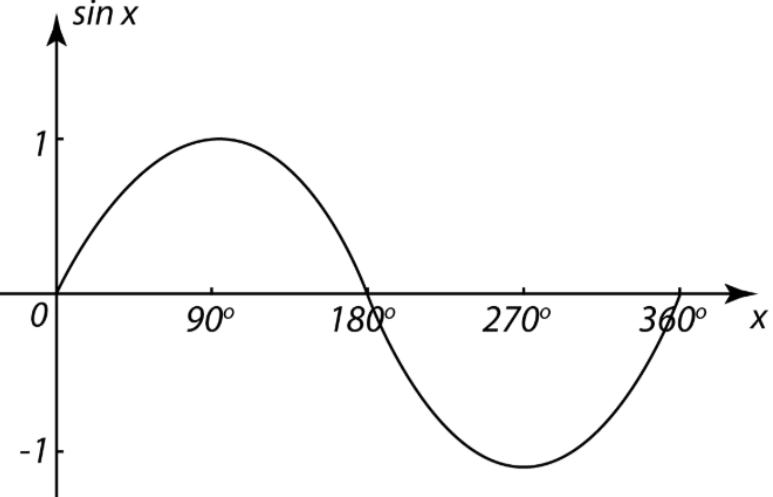QuestionAnswers

# Find the area bounded by the curve y=sin x between x=0 and x=2$\pi$.Verified
149.1k+ views
Hint: To find the area bounded by the given curve, observe the graph of y=sin x from the limit given above, there are 2 areas whose sum will give the total area.

The graph of y=sin x is shown below:Therefore, the required area will be the sum of area under curve ${0^ \circ }\, to \,{180^ \circ }$ and the area under curve ${180^ \circ }\, to \,{360^ \circ }$.
Therefore,
$\Rightarrow \int\limits_0^\pi {\sin \,x\,dx} + |\int\limits_\pi ^{2\pi } {\sin \,x\,dx} |$
On integrating, we get,
$\Rightarrow \left[ { - \cos \,x} \right]_0^\pi + |\left[ { - \cos x} \right]_\pi ^{2\pi }|$
Let us apply the limits,
$\Rightarrow \left[ { - \cos \,\pi + \cos 0} \right] + |\left[ { - \cos \,2\pi + \cos \pi } \right]|$
Let us put the values in the above equation, we get,
$\Rightarrow 1 + 1 + |\left[ { - 1 - 1} \right]|$
On simplifying the above equation, we get,
$\Rightarrow 2 + 2$
Answer $\Rightarrow 4\,sq.\,units$

Note: Make sure that you take the mod in the lower curve part because area can’t be negative. Separate the integration over two periods.